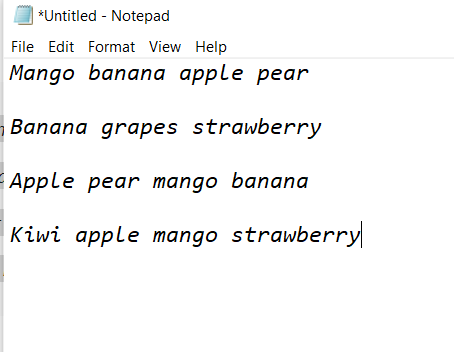# Python program to capitalize the first letter of every word in the file

The following article contains programs to read a file and capitalize the first letter of every word in the file and print it as output. To capitalize the first letter we will use different methods using Python. The Python String Method is used to convert the first character in each word to Uppercase and the remaining characters to Lowercase in the string and return the new string.

Examples:

```Input: hello world
Output: Hello World```

### Input text:temp.txt

### Method 1:  Capitalize First Letter in Python using title()

Here, we are using the Python loop, and with the help of the title() function to capitalize each word.

## Python3

 `file_gfg ``=` `open``(``'temp.txt'``, ``'r'``)`   `for` `line ``in` `file_gfg:` `    ``output ``=` `line.title()` `    ``print``(output)`

Output:

`Mango Banana Apple Pear Banana Grapes Strawberry Apple Pear Mango Banana Kiwi Apple Mango Strawberry`

### Method 2:  Capitalize First Letter in Python using capitalize()

Here, we are using the Python loop, and with the help of the capitalize() function to capitalize each word.

## Python3

 `result ``=` `""   ` `file_gfg ``=` `open``(``'temp.txt'``, ``'r'``)` `for` `line ``in` `file_gfg:` `    ``g ``=` `line.split()` `    ``for` `elem ``in` `g:` `        ``# capitalize first letter of each word and add to a string` `        ``if` `len``(result) > ``0``:` `            ``result ``=` `result ``+` `" "` `+` `elem.strip().capitalize()` `        ``else``:` `            ``result ``=` `elem.capitalize()`   `print``(result)`

Output:

`Mango Banana Apple Pear Banana Grapes Strawberry Apple Pear Mango Banana Kiwi Apple Mango Strawberry`

### Method 3:  Capitalize First Letter in Python using capwords()

Here, we are using the Python loop, and with the help of the capwords() function to capitalize each word.

## Python3

 `import` `string`   `result1 ``=` `""   ` `file_gfg ``=` `open``(``'temp.txt'``, ``'r'``)` `for` `line ``in` `file_gfg:` `    ``g ``=` `line.split()` `    ``for` `elem ``in` `g:` `        ``# capitalize first letter of each word and add to a string` `        ``if` `len``(result1) > ``0``:` `            ``result1 ``=` `result1 ``+` `" "` `+` `string.capwords(elem)` `        ``else``:` `            ``result1 ``=` `string.capwords(elem)`   `print``(result1)`

Output:

`Mango Banana Apple Pear Banana Grapes Strawberry Apple Pear Mango Banana Kiwi Apple Mango Strawberry`

### Method 4:  Capitalize First Letter in Python using regex

Here, we are using the Python loop, and with the help of the regex to capitalize each word.

## Python3

 `import` `re` `def` `convert_to_uupercase(m):` `    ``return` `m.group(``1``) ``+` `m.group(``2``).upper()`   `result ``=` `""   ` `file_gfg ``=` `open``(``'temp.txt'``, ``'r'``)` `for` `line ``in` `file_gfg:` `    ``g ``=` `line.split()` `    ``for` `elem ``in` `g:` `        ``# capitalize first letter of each word and add to a string` `        ``if` `len``(result) > ``0``:` `            ``result ``=` `result ``+` `re.sub(``"(^|\s)(\S)"``, convert_to_uupercase, elem) ``+` `" "` `        ``else``:` `            ``result ``=` `re.sub(``"(^|\s)(\S)"``, convert_to_uupercase, elem)`   `print``(result)`

Output:

`Mango Banana Apple Pear Banana Grapes Strawberry Apple Pear Mango Banana Kiwi Apple Mango Strawberry`

Whether you're preparing for your first job interview or aiming to upskill in this ever-evolving tech landscape, GeeksforGeeks Courses are your key to success. We provide top-quality content at affordable prices, all geared towards accelerating your growth in a time-bound manner. Join the millions we've already empowered, and we're here to do the same for you. Don't miss out - check it out now!

Previous
Next